Question

# Determine the molecular mass and its uncertainty for C6H5OH . Significant figures are graded for this...

Determine the molecular mass and its uncertainty for C6H5OH . Significant figures are graded for this problem. To avoid errors, do not round your answers until the very end of your calculations and use the atomic masses given in the table.

Element Atomic Mass
H 1.00794 ± 0.00007
C 12.0107 ± 0.0008
O 15.9994 ± 0.0003

Given compound = C6H5OH

Molecular mass of H = 1.00794 ± 0.00007

Molecular mass of C = 12.0107 ± 0.0008

Molecular mass of O = 15.9994 ± 0.0003

Molecular mass of C6H5OH

= 6*Molecular mass of C + (5+1)*Molecular mass of H + 1*Molecular mass of O

= 6*(12.0107 ± 0.0008) + (5+1)*(1.00794 ± 0.00007) + 1*(15.9994 ± 0.0003)

= (72.0642 ± 0.0048) + (6.04764 ± 0.00042) + (15.9994 ± 0.0003)

= 94.11124 ± 0.00552

= 94.1112 ± 0.005

#### Earn Coins

Coins can be redeemed for fabulous gifts.

Similar Homework Help Questions
• ### Determine the molecular mass and its uncertainty for C3H7OH. Note: Significant figures are graded for this...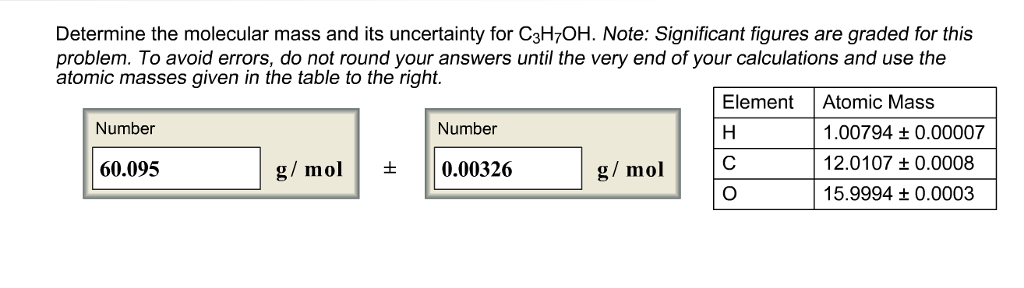Determine the molecular mass and its uncertainty for C3H7OH. Note: Significant figures are graded for this problem. To avoid errors, do not round your answers until the very end of your calculations and use the atomic masses given in the table to the right. ElementAtomic Mass Number Number .00794 t 0.00007 12.0107 t0.0008 15.9994 t 0.0003 60.095 g/mol+0.00326 g/ mol O

• ### Remember your significant figures! Use the following molecular weights: C = 12.0107 H = 1.00794 O...

Remember your significant figures! Use the following molecular weights: C = 12.0107 H = 1.00794 O = 15.9994 N = 14.00674 Fe = 55.845 S = 32.06 Co = 58.93 Na = 22.9898 Question 1: Your molecular weights are given above, where would you find these if they were not given? 1 mark

• ### A solution of HNO, is standardized by reaction with pure sodium carbonate. 2H Na,CO, » 2...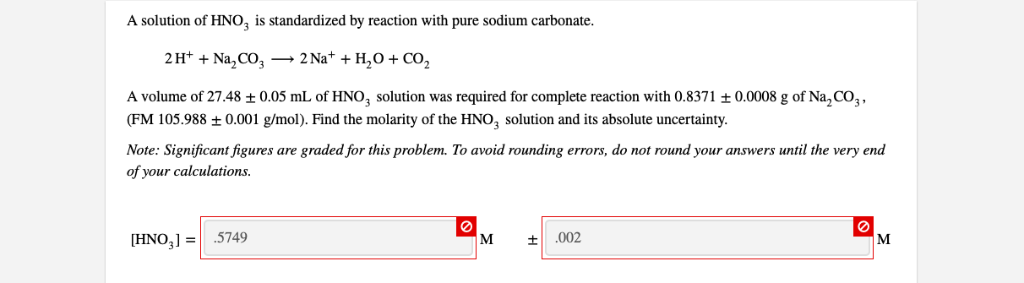A solution of HNO, is standardized by reaction with pure sodium carbonate. 2H Na,CO, » 2 Na* +H,O + CO2 A volume of 27.48 ± 0.05 mL of HNO3 solution was required for complete reaction with 0.8371 ± 0.0008 g of Na,CO3, (FM 105.988 0.001 g/mol). Find the molarity of the HNO solution and its absolute uncertainty Note: Significant figures are graded for this problem. To avoid rounding errors, do not round your answers until the very end of your...

• ### Perform the following calculations and determine the absolute and percent relative uncertainty. Express each answer with...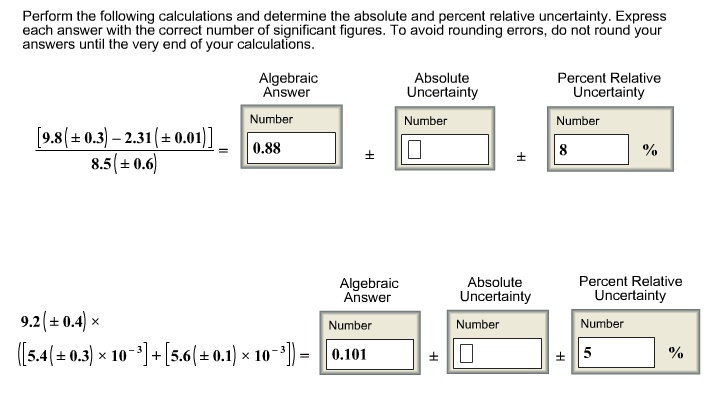Perform the following calculations and determine the absolute and percent relative uncertainty. Express each answer with the correct number of significant figures. To avoid rounding errors, do not round your answers until the very end of your calculations. Perform the following calculations and determine the absolute and percent relative uncertainty. Express each answer with the correct number of significant figures. To avoid rounding errors, do not round your answers until the very end of your calculations Absolute Uncertainty Number Percent...

• ### A solution of HNO is standardized by reaction with pure sodium carbonate 2H+ + Na,CO3-2 Na+ + H2O + CO2 A volume of 25....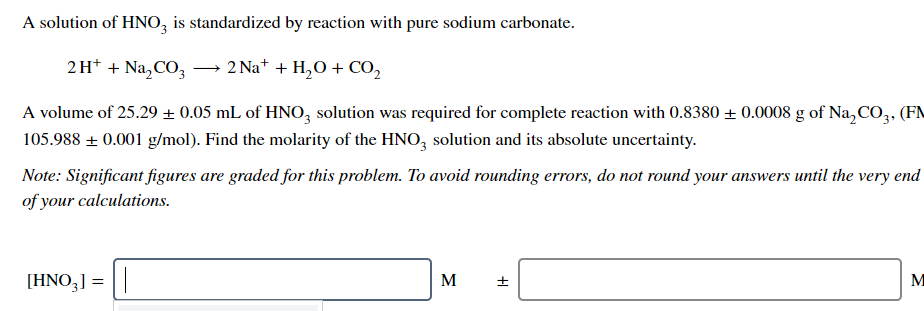A solution of HNO is standardized by reaction with pure sodium carbonate 2H+ + Na,CO3-2 Na+ + H2O + CO2 A volume of 25.29 + 0.05 mL of HNO3 solution was required for complete reaction with 0.8380+0.0008 g of Na,CO3. (FN 105.988 0.001 g/mol). Find the molarity of the HNO3 solution and its absolute uncertainty. Note: Significant figures are graded for this problem. To avoid rounding errors, do not round your answers until the very end of your calculations IHNO,I-I...

• ### A solution of F− is prepared by dissolving 0.0722±0.0005 g NaF (molar mass = 41.989±0.001 g/mol)...

A solution of F− is prepared by dissolving 0.0722±0.0005 g NaF (molar mass = 41.989±0.001 g/mol) in 154.00±0.06 mL of water. Calculate the concentration of F− in solution and its absolute uncertainty. Significant figures are graded for this problem. To avoid rounding errors, do not round your answers until the very end of your calculations.

• ### A solution of F− is prepared by dissolving 0.0986±0.0004 g NaF (molar mass = 41.989±0.001 g/mol...

A solution of F− is prepared by dissolving 0.0986±0.0004 g NaF (molar mass = 41.989±0.001 g/mol ) in 162.00±0.06 mL of water. Calculate the concentration of F− in solution and its absolute uncertainty. Significant figures are graded for this problem. To avoid rounding errors, do not round your answers until the very end of your calculations.

• ### A solution of HNO3 is standardized by reaction with pure sodium carbonate. 2H++Na2CO3⟶2Na++H2O+CO2 A volume of...

A solution of HNO3 is standardized by reaction with pure sodium carbonate. 2H++Na2CO3⟶2Na++H2O+CO2 A volume of 28.52±0.05 mL of HNO3 solution was required for complete reaction with 0.8605±0.0008 g of Na2CO3 , (FM 105.988±0.001 g/mol ). Find the molarity of the HNO3 solution and its absolute uncertainty. Note: Significant figures are graded for this problem. To avoid rounding errors, do not round your answers until the very end of your calculations.

• ### A solution of HNO2 is standardized by reaction with pure sodium carbonate. 2 Na* H20 2H Na2C03 - CO2 A volume of 26.18...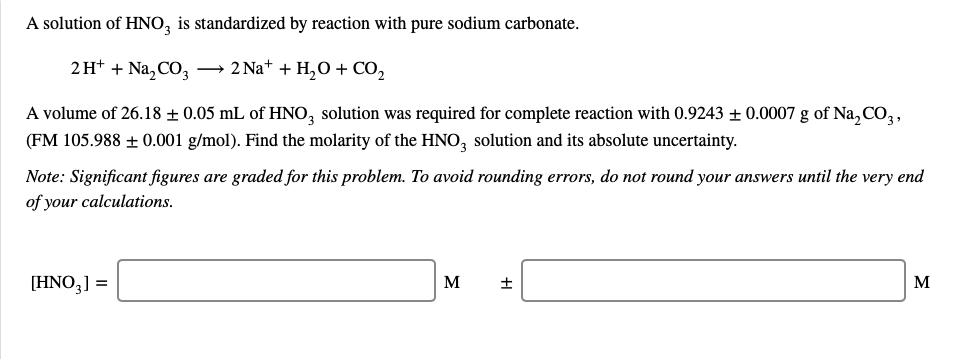A solution of HNO2 is standardized by reaction with pure sodium carbonate. 2 Na* H20 2H Na2C03 - CO2 A volume of 26.18 ± 0.05 mL of HNO2 solution was required for complete reaction with 0.9243 ± 0.0007 g of Na,CO3, (FM 105.988 0.001 g/mol). Find the molarity of the HNO2 solution and its absolute uncertainty Note: Significant figures are graded for this problem. To avoid rounding errors, do not round your answers until the very end of your calculations...

• ### of15 > Attempt A solution of HNO, is standardized by reaction with pure sodium carbonate. A...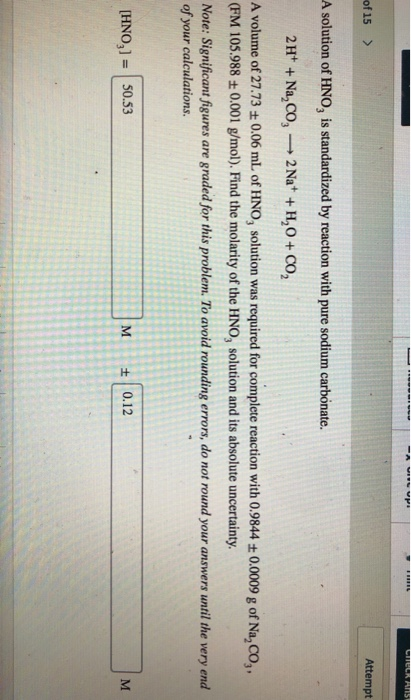of15 > Attempt A solution of HNO, is standardized by reaction with pure sodium carbonate. A volume of 27.73 t 0.06 mL of HNO, solution was required for complete reaction with 0.9844t 0.0009 g of Na,Co,, (FM 105.988 t0.001 g/mol). Find the molarity of the HNO, solution and its absolute uncertainty. Note: Significant figures are graded for this problem. To avoid rounding errors, do not round your answers until the very end of your calculations. HNO,]50.53 M 0.12